# Pandas数据可视化的备忘录

2020/11/12 23:28Pandas本身可以在后端使用Matplotlib并为你呈现可视化效果。它使得使用数据帧列绘制图变得非常容易。Pandas使用比Matplotlib更高级别的API。因此，它可以用更少的代码行来绘制绘图。

pip install pandas


conda install pandas


### pandas可视化

#### 直线图

import pandas as pd
a = pd.Series([40, 34, 30, 22, 28, 17, 19, 20, 13, 9, 15, 10, 7, 3])
a.plot()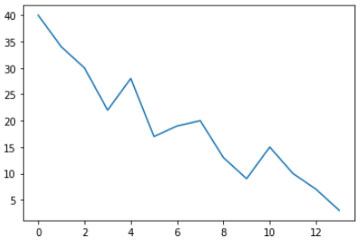a.plot(figsize=(8, 6), color='green', title = 'Line Plot', fontsize=12)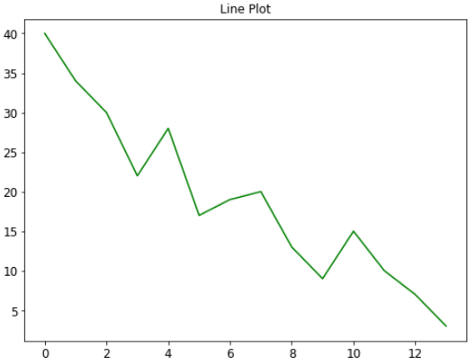#### 面积图

a.plot(kind='area')


a.plot.area()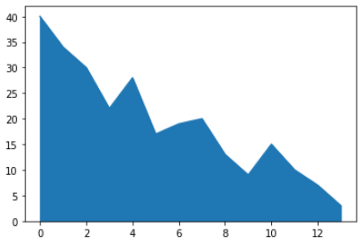b = pd.Series([45, 22, 12, 9, 20, 34, 28, 19, 26, 38, 41, 24, 14, 32])
c = pd.Series([25, 38, 33, 38, 23, 12, 30, 37, 34, 22, 16, 24, 12, 9])
d = pd.DataFrame({'a':a, 'b': b, 'c': c})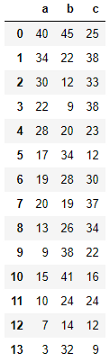d.plot.area(figsize=(8, 6), title='Area Plot')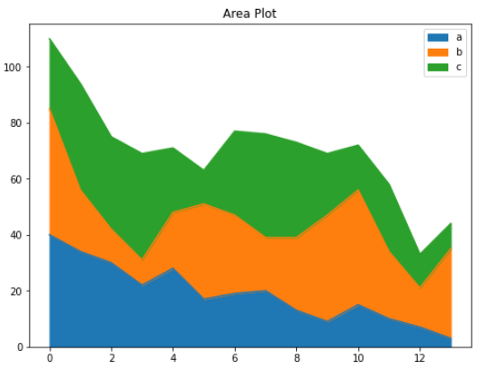d.plot.area(alpha=0.4, color=['coral', 'purple', 'lightgreen'],figsize=(8, 6), title='Area Plot', fontsize=12)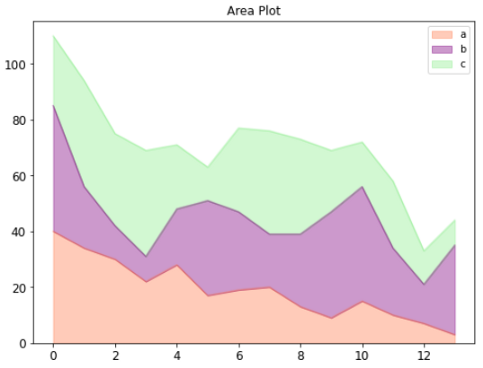“alpha”参数为绘图添加了一些半透明的外观。

**plot()**可以执行11种类型的绘图：

1. line
2. area
3. bar
4. barh
5. pie
6. box
7. hexbin
8. hist
9. kde
10. density
11. scatter

df = pd.read_csv('nhanes_2015_2016.csv')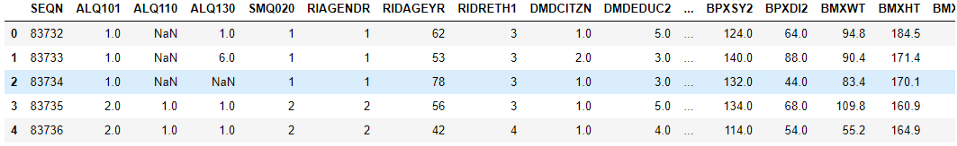df.columns


Index(['SEQN', 'ALQ101', 'ALQ110', 'ALQ130', 'SMQ020', 'RIAGENDR', 'RIDAGEYR', 'RIDRETH1', 'DMDCITZN', 'DMDEDUC2', 'DMDMARTL', 'DMDHHSIZ', 'WTINT2YR', 'SDMVPSU', 'SDMVSTRA', 'INDFMPIR', 'BPXSY1', 'BPXDI1', 'BPXSY2', 'BPXDI2', 'BMXWT', 'BMXHT', 'BMXBMI', 'BMXLEG', 'BMXARML', 'BMXARMC', 'BMXWAIST', 'HIQ210', 'DMDEDUC2x', 'DMDMARTLx'], dtype='object')


#### 直方图

df['BMXWT'].hist()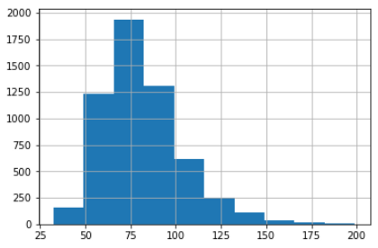df[['BMXWT', 'BMXHT', 'BMXBMI']].plot.hist(stacked=True, bins=20, fontsize=12, figsize=(10, 8))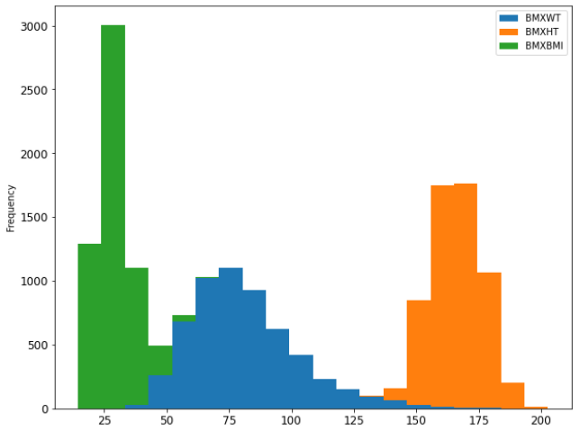df[['BMXWT', 'BMXHT', 'BMXBMI']].hist(bins=20,figsize=(10, 8))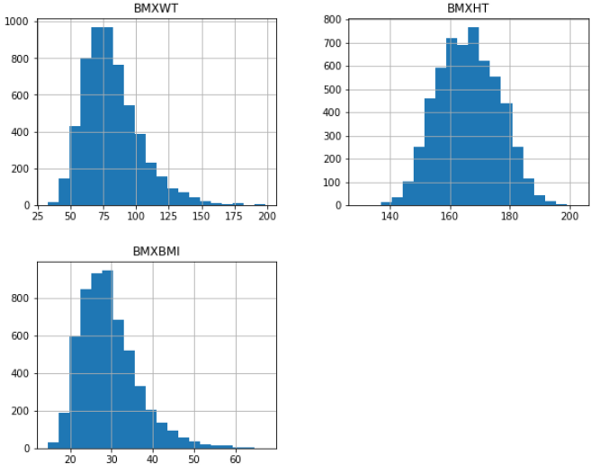df["DMDEDUC2x"] = df.DMDEDUC2.replace({1: "less than 9", 2: "9-11", 3: "HS/GED", 4: "Some college/AA", 5: "College", 7: "Refused", 9: "Don't know"})


df[['DMDEDUC2x', 'BPXSY1']].hist(by='DMDEDUC2x', figsize=(18, 12))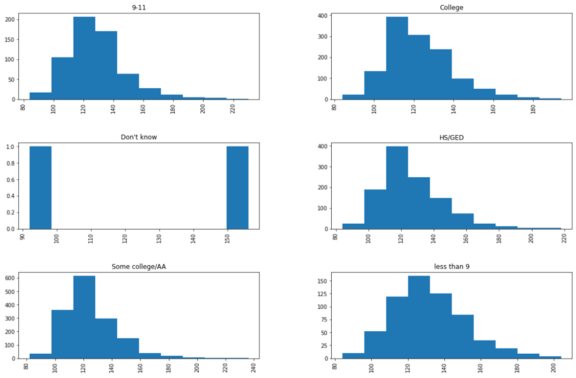#### 条形图

df["DMDMARTLx"] = df.DMDMARTL.replace({1: "Married", 2: "Widowed", 3: "Divorced", 4: "Separated", 5: "Never married", 6: "Living w/partner", 77: "Refused"})


df.groupby('DMDMARTLx')['BPXSY1'].mean().plot(kind='bar', rot=45, fontsize=10, figsize=(8, 6))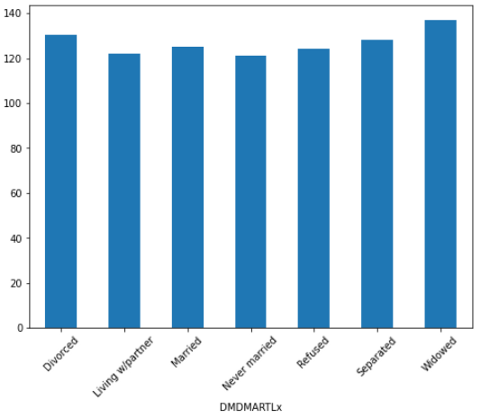df.groupby('DMDEDUC2x')['BPXSY1'].mean().plot(kind='barh', rot=45, fontsize=10, figsize=(8, 6))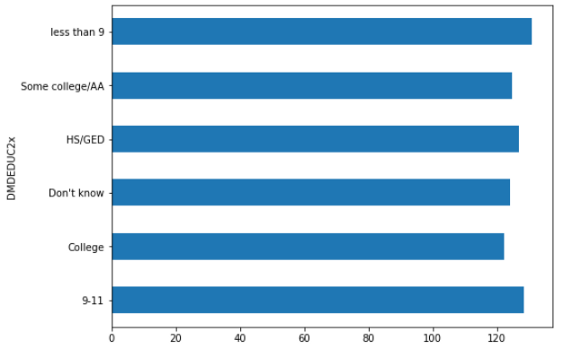df_bmx = df.groupby('RIDRETH1')['BMXWT', 'BMXHT', 'BMXBMI'].mean().reset_index()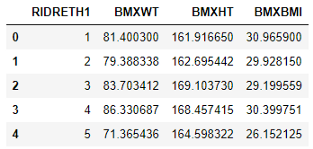df_bmx.plot(x = 'RIDRETH1',
y=['BMXWT', 'BMXHT', 'BMXBMI'],
kind = 'bar',
color = ['lightblue', 'red', 'yellow'],
fontsize=10)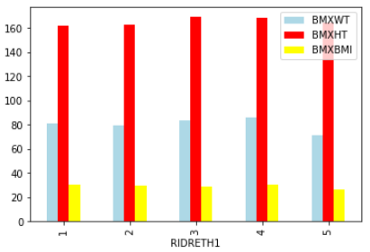df_bmx.plot(x = 'RIDRETH1',
y=['BMXWT', 'BMXHT', 'BMXBMI'],
kind = 'bar', stacked=True,
color = ['lightblue', 'red', 'yellow'],
fontsize=10)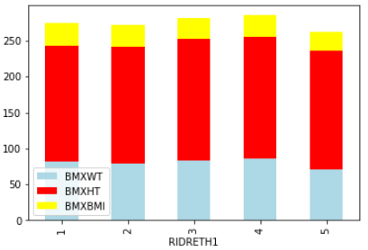#### 饼图

df_edu_marit = df.groupby('DMDEDUC2x')['DMDMARTL'].count()
pd.Series(df_edu_marit)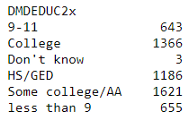ax = pd.Series(df_edu_marit).plot.pie(subplots=True, label='',
labels = ['College Education', 'high school',
'less than high school', 'Some college',
'HS/GED', 'Unknown'],
figsize = (8, 6),
colors = ['lightgreen', 'violet', 'coral', 'skyblue', 'yellow', 'purple'], autopct = '%.2f')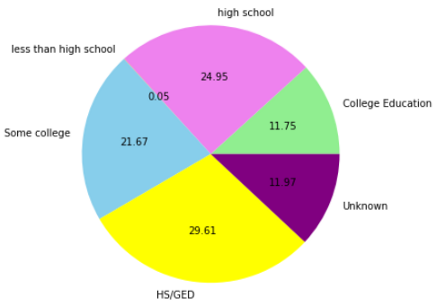#### 箱线图

color = {'boxes': 'DarkBlue', 'whiskers': 'coral',
'medians': 'Black', 'caps': 'Green'}
df[['BMXBMI', 'BMXLEG', 'BMXARML']].plot.box(figsize=(8, 6),color=color)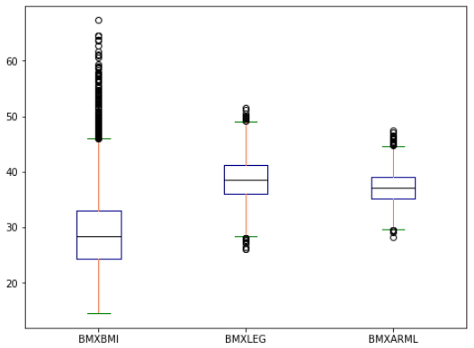#### 散点图

df.head(300).plot(x='BMXBMI', y= 'BPXSY1', kind = 'scatter')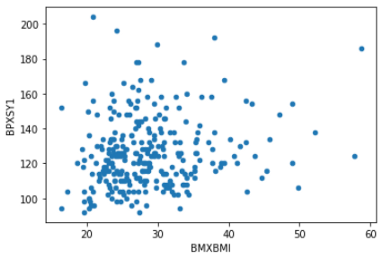df.head(500).plot.scatter(x= 'BMXWT', y = 'BMXHT', c ='BMXLEG', s=50, figsize=(8, 6))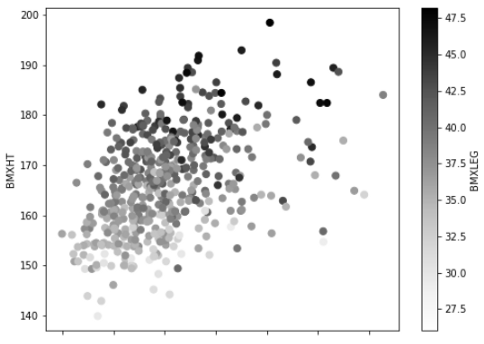df.head(200).plot.scatter(x= 'BMXHT', y = 'BMXWT',
s =df['BMXBMI'][:200] * 7,
alpha=0.5, color='purple',
figsize=(8, 6))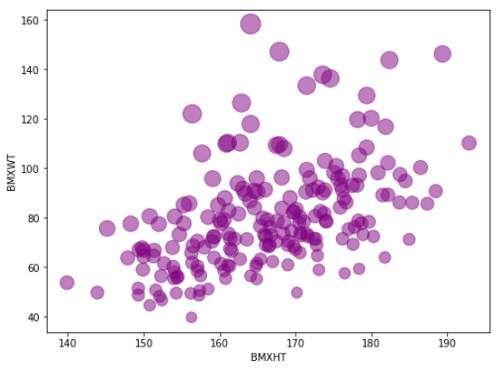#### 六边形

df.plot.hexbin(x='BMXARMC', y='BMXLEG', gridsize= 20)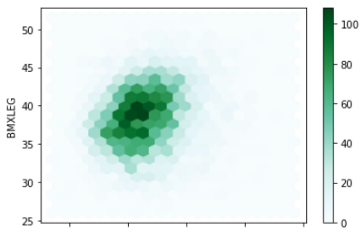df.plot.hexbin(x='BMXARMC', y='BMXLEG', C = 'BMXHT',
reduce_C_function=np.max,
gridsize=15,
figsize=(8,6))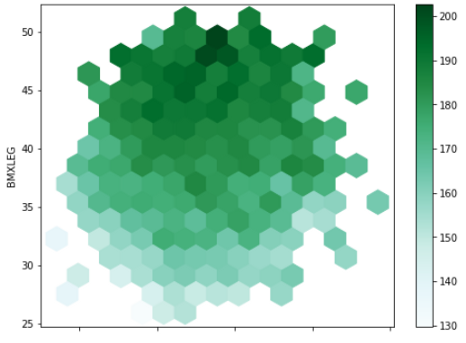df.plot.hexbin(x='BMXARMC', y='BMXLEG', C = 'BMXHT',
reduce_C_function=np.max,
gridsize=15,
figsize=(8,6),
cmap = 'viridis')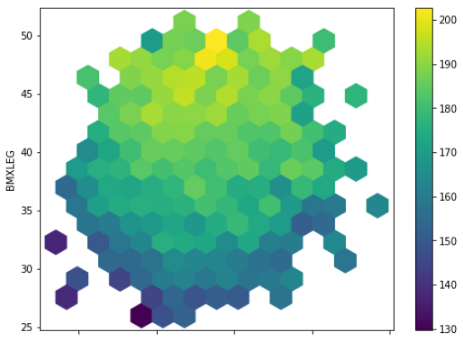### 一些高级可视化

#### 散点矩阵

from pandas.plotting import scatter_matrix

scatter_matrix(df[['BMXWT', 'BMXHT', 'BMXBMI', 'BMXLEG', 'BMXARML']], alpha = 0.2, figsize=(10, 8), diagonal = 'kde')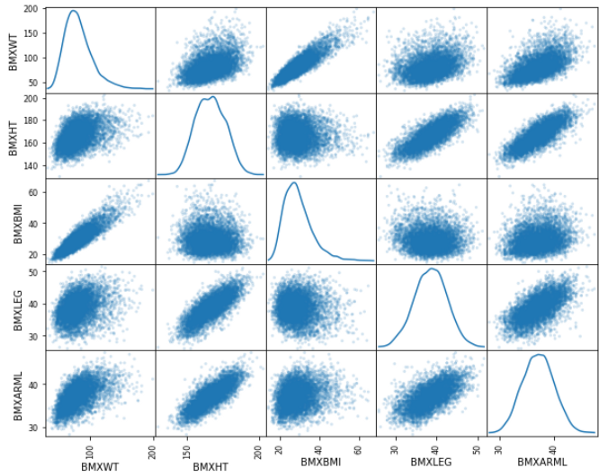#### KDE或密度图

df['BMXWT'].plot.kde()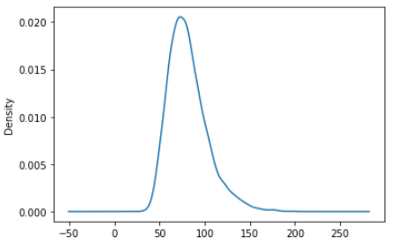df[['BMXWT', 'BMXHT', 'BMXBMI']].plot.kde(figsize = (8, 6))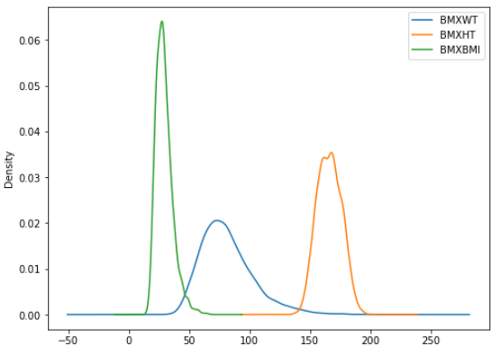#### Parallel_coordinates

from pandas.plotting import parallel_coordinates

parallel_coordinates(df[['BMXWT', 'BMXHT', 'BMXBMI', 'RIAGENDR']].dropna().head(200), 'RIAGENDR', color=['blue', 'violet'])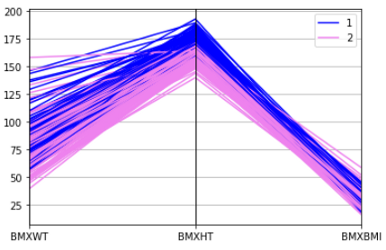#### Bootstrap_plot

from pandas.plotting import bootstrap_plot

bootstrap_plot(df['BMXBMI'], size=100, samples=1000, color='skyblue')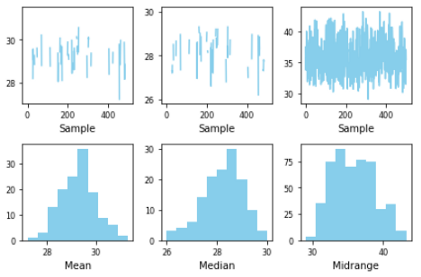### 结论

sklearn机器学习中文官方文档： http://sklearn123.com/0 评论
0 收藏
0